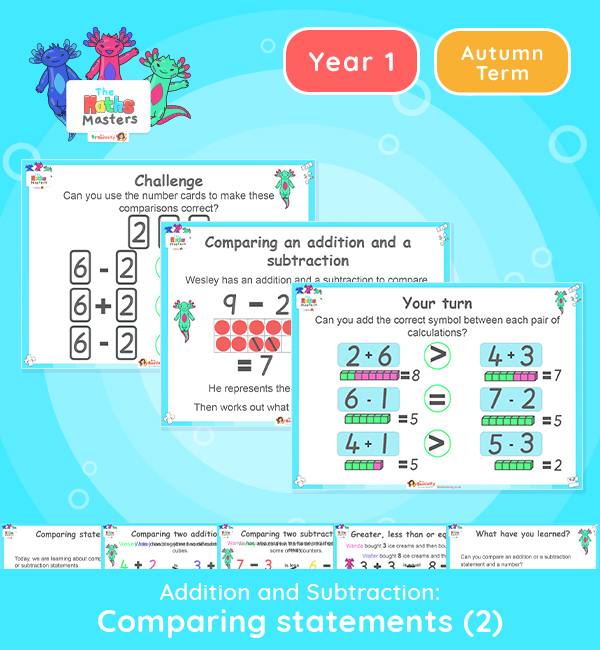# Year 1 | Comparing Addition and Subtraction Statements Part 2 Lesson Presentation## White Rose year 1 addition and subtraction resources

Aligned with the White Rose Maths scheme of work, this Year 1 | Comparing Addition and Subtraction Statements Part 2 Lesson Presentation is fully editable and designed for the Year 1 maths curriculum to cover the following curriculum objectives:

This is the second of two lessons on using inequality symbols and using them to compare two addition or subtraction statements .

White Rose Maths Small Steps: Comparing addition and subtraction statements a + b > c + d

NC Links: Read, write and interpret mathematical statements involving addition (+), subtraction (-), and equals (=) signs. • Add and subtract one digit numbers to 10, including zero. • Solve one step problems that involve addition and subtraction using concrete objects and pictorial representations.  • Identify and represent numbers using objects and pictorial representations including the number line, and use the language of: equal to, more than, less than (fewer), most, least.

Ready-to-progress criteria:  Previous Experience: Devise and record number stories, using pictures, numbers and symbols.

1AS-2 Read, write and interpret equations continuing addition, subtraction  and equals symbols, and relate additive expressions and equations to real-life contexts.

1NPV-2 Reason about the location of numbers to 20 within the linear number system, including using < > and =

Future applications: Represent compositions and decomposition of numbers using equations.

TAF Statements: Working Towards Add and subtract (one digit numbers) explaining their method verbally in pictures or using apparatus.

Greater Depth: Use reasoning about numbers and relationships to solve more complex problems and explain their thinking.

Explore our other year 1 addition and subtraction resources.

Free

#### Recently Viewed